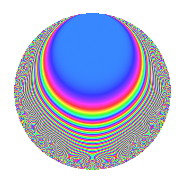# Properties

 Label 619.2.a.aLevel 619 Weight 2 Character orbit 619.a Self dual Yes Analytic conductor 4.943 Analytic rank 1 Dimension 21 CM No

# Related objects

## Newspace parameters

 Level: $$N$$ = $$619$$ Weight: $$k$$ = $$2$$ Character orbit: $$[\chi]$$ = 619.a (trivial)

## Newform invariants

 Self dual: Yes Analytic conductor: $$4.94273988512$$ Analytic rank: $$1$$ Dimension: $$21$$ Fricke sign: $$1$$ Sato-Tate group: $\mathrm{SU}(2)$

## $q$-expansion

The dimension is sufficiently large that we do not compute an algebraic $$q$$-expansion, but we have computed the trace expansion.

 $$\operatorname{Tr}(f)(q) =$$ $$21q - 9q^{2} - 5q^{3} + 15q^{4} - 21q^{5} - 6q^{6} - 4q^{7} - 21q^{8} + 6q^{9} + O(q^{10})$$ $$\operatorname{Tr}(f)(q) =$$ $$21q - 9q^{2} - 5q^{3} + 15q^{4} - 21q^{5} - 6q^{6} - 4q^{7} - 21q^{8} + 6q^{9} + q^{10} - 27q^{11} - 8q^{12} - 11q^{13} - 19q^{14} - 10q^{15} + 11q^{16} - 14q^{17} - 14q^{18} - 15q^{19} - 25q^{20} - 42q^{21} + 12q^{22} - 14q^{23} - 8q^{24} + 16q^{25} - 11q^{26} - 5q^{27} + q^{28} - 78q^{29} + q^{30} - 8q^{31} - 41q^{32} - 6q^{33} + 7q^{34} - 3q^{35} - q^{36} - 23q^{37} + 21q^{38} - 4q^{39} + 12q^{40} - 59q^{41} + 39q^{42} + 2q^{43} - 50q^{44} - 36q^{45} - 15q^{46} - 12q^{47} + 10q^{48} + 17q^{49} - 23q^{50} - 8q^{51} + 18q^{52} - 36q^{53} - 4q^{54} + 23q^{55} - 28q^{56} - 24q^{57} + 46q^{58} - 17q^{59} + 8q^{60} - 22q^{61} + 42q^{62} - 6q^{63} + 49q^{64} - 53q^{65} + 29q^{66} + 15q^{67} - 16q^{68} - 30q^{69} + 44q^{70} - 56q^{71} + 12q^{72} - 2q^{73} - 12q^{74} + 2q^{75} - 4q^{76} - 47q^{77} + 36q^{78} + 5q^{79} + 15q^{80} - 19q^{81} + 47q^{82} - q^{83} - 20q^{84} - 29q^{85} - 23q^{86} + 44q^{87} + 61q^{88} - 12q^{89} + 91q^{90} + 5q^{91} + 35q^{92} - 15q^{93} + 34q^{94} - 17q^{95} + 14q^{96} + 21q^{97} + 24q^{98} - 38q^{99} + O(q^{100})$$

## Embeddings

For each embedding $$\iota_m$$ of the coefficient field, the values $$\iota_m(a_n)$$ are shown below.

For more information on an embedded modular form you can click on its label.

Label $$a_{2}$$ $$a_{3}$$ $$a_{4}$$ $$a_{5}$$ $$a_{6}$$ $$a_{7}$$ $$a_{8}$$ $$a_{9}$$ $$a_{10}$$
1.1 −2.73280 −1.70616 5.46819 2.03444 4.66258 3.83859 −9.47786 −0.0890335 −5.55971
1.2 −2.61324 2.79918 4.82902 −2.04890 −7.31494 −3.26475 −7.39292 4.83543 5.35427
1.3 −2.53656 −1.36544 4.43411 −3.55564 3.46351 0.675306 −6.17426 −1.13558 9.01908
1.4 −2.32447 0.941827 3.40317 −2.38281 −2.18925 2.74500 −3.26162 −2.11296 5.53878
1.5 −2.08256 0.916211 2.33704 2.62373 −1.90806 −1.16947 −0.701915 −2.16056 −5.46408
1.6 −1.67412 −2.96683 0.802676 −4.06102 4.96682 1.39415 2.00446 5.80206 6.79864
1.7 −1.42511 −0.995739 0.0309400 2.82686 1.41904 −2.89800 2.80613 −2.00850 −4.02859
1.8 −1.37173 0.756788 −0.118367 −1.92672 −1.03811 −0.911742 2.90582 −2.42727 2.64293
1.9 −0.887456 −2.66181 −1.21242 −0.744827 2.36224 −0.702395 2.85088 4.08525 0.661001
1.10 −0.788010 1.45313 −1.37904 −3.12358 −1.14508 4.52894 2.66272 −0.888427 2.46141
1.11 −0.723996 3.01745 −1.47583 −2.02994 −2.18462 −2.86695 2.51649 6.10499 1.46966
1.12 −0.691014 −0.832025 −1.52250 1.28552 0.574940 1.22773 2.43410 −2.30773 −0.888311
1.13 0.152908 1.46557 −1.97662 0.468718 0.224098 −3.63648 −0.608058 −0.852102 0.0716709
1.14 0.273610 −2.79069 −1.92514 −0.828377 −0.763560 5.25882 −1.07396 4.78794 −0.226652
1.15 0.642946 1.06499 −1.58662 −0.849273 0.684732 −0.754832 −2.30600 −1.86579 −0.546037
1.16 1.15106 −1.36322 −0.675056 1.59267 −1.56915 −0.851998 −3.07916 −1.14163 1.83326
1.17 1.32477 0.433395 −0.244976 −1.11370 0.574150 −1.01156 −2.97408 −2.81217 −1.47540
1.18 1.53518 1.64763 0.356779 −4.31073 2.52940 −5.09714 −2.52264 −0.285328 −6.61776
1.19 1.61466 −2.35583 0.607120 0.981279 −3.80386 −0.0820085 −2.24902 2.54995 1.58443
1.20 1.75261 −0.558565 1.07166 −3.69018 −0.978948 2.79513 −1.62703 −2.68801 −6.46747
See all 21 embeddings
 $$n$$: e.g. 2-40 or 990-1000 Embeddings: e.g. 1-3 or 1.21 Significant digits: Format: Complex embeddings Normalized embeddings Satake parameters Satake angles

## Inner twists

This newform does not have CM; other inner twists have not been computed.

## Atkin-Lehner signs

$$p$$ Sign
$$619$$ $$1$$

## Hecke kernels

This newform can be constructed as the kernel of the linear operator $$T_{2}^{21} + \cdots$$ acting on $$S_{2}^{\mathrm{new}}(\Gamma_0(619))$$.